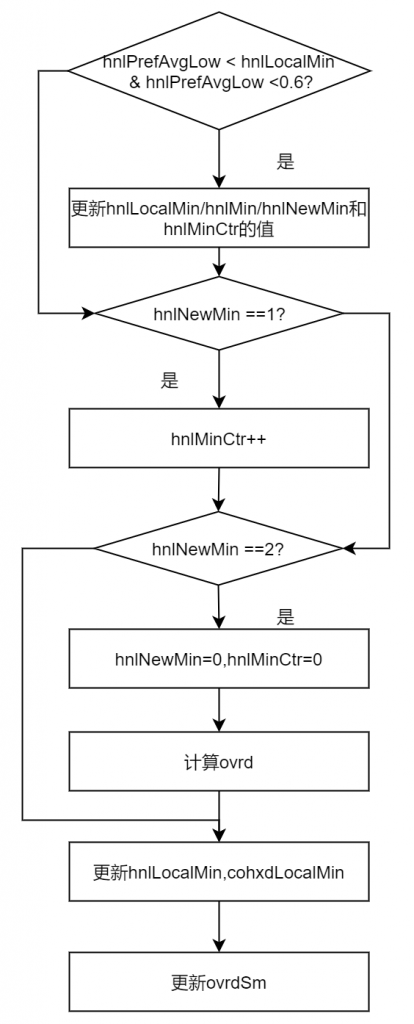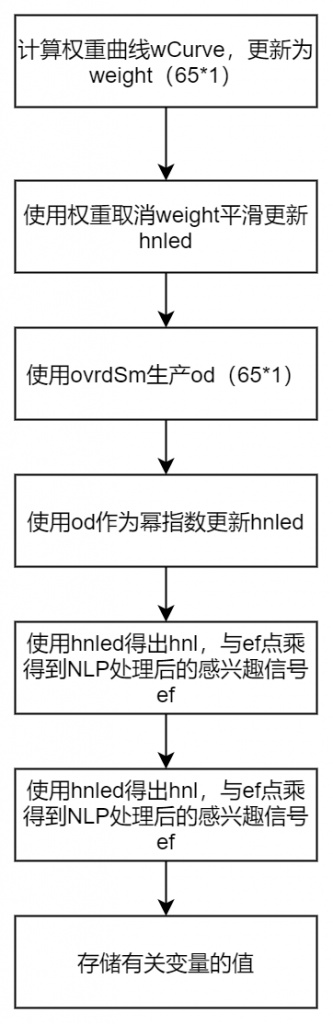## 非线性处理部分

hnlLocalMin是对hnlPrefAvgLow的最小值跟踪，其初始值为1，在满足了下面判断条件下，实际上就是发现了更小的值（并且这个最小值符合条件），就会对其更新，然后将hnlNewMin设置为1，hnlMinCtr重新置0。

if hnlPrefAvgLow < hnlLocalMin & hnlPrefAvgLow < 0.6
hnlLocalMin = hnlPrefAvgLow;
hnlMin = hnlPrefAvgLow;
hnlNewMin = 1;
hnlMinCtr = 0;
end
if hnlNewMin == 1:
hnlMinCtr = hnlMinCtr + 1;
end
if hnlMinCtr == 2:
hnlNewMin = 0;
hnlMinCtr = 0;
ovrd = max(log(0.00000001)/log(hnlMin +1e-10),3);
end

hnlLocalMin = min(hnlLocalMin + 0.0008/mult, 1);
cohxdLocalMin = min(cohxdLocalMin + 0.0004/mult, 1);

if ovrd < ovrdSm
ovrdSm = 0.99*ovrdSm + 0.01*ovrd;
else
ovrdSm = 0.9*ovrdSm + 0.1*ovrd;
endekEn = sum(Se);
dkEn = sum(Sd);
if divergeState == 0
if ekEn > dkEn
ef = df;
divergeState = 1;
end
else
if ekEn*1.05 < dkEn
divergeState = 0;
else
ef = df;
end
end

if ekEn > dkEn*19.95
WFb=zeros(N+1,M); % Block-based FD NLMS
end

ekEnV(kk) = ekEn;
dkEnV(kk) = dkEn;
hnlLocalMinV(kk) = hnlLocalMin;
cohxdLocalMinV(kk) = cohxdLocalMin;
hnlMinV(kk) = hnlMin;aggFact = 0.3;
wCurve = [0; aggrFact*sqrt(linspace(0,1,N))’ + 0.1];
weight = wCurve;
hnled = weight.*min(hnlPrefAvg, hnled) + (1 – weight).*hnled;

od = ovrdSm *sqrt(linspace(0,1,N+1))’ + 1;
sshift = ones(N+1,1);
hnled = hnled.^(od.*sshift);

hnl=hnled;
ef = ef.*(hnl);
ovrdV(kk) = ovrdSm;
hnledAvg(kk) = 1-mean(1-cohed(echoBandRange));
hnlxdAvg(kk) = 1-mean(cohxd(echoBandRange));
hnlSortQV(kk) = hnlPrefAvgLow;
hnlPrefAvgV(kk) = hnlPrefAvg;